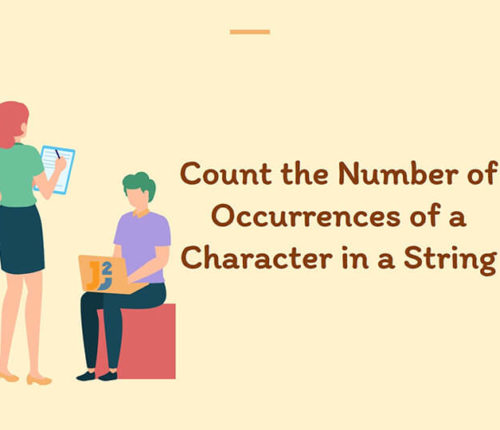# java program to check prime number

This is easy question. It may not be asked you directly, but you can use this program to create many other complex program.
A prime number is a natural number greater than 1 that has no positive divisors other than 1 and itself.

#### Program logic:

If any number which is not divisible by any other number which is less than or equal to square root of that number, then it is prime number.
Lets create java program for it:

Run above program, you will get following output:

Please go through java interview programs for more such programs.

import_contacts

### You may also like:## Related Posts

•05 April

### Count the Number of Occurrences of a Character in a String in Java

In this article, we will look at a problem: Given an Input String and a Character, we have to Count the Occurrences of such Character in the given String. For Example, If the Given String is : "Java2Blog" and we have to count the occurrences of Character ‘a’ in the String. Therefore, Count of 'a' […]

•24 December

### How to capitalize first letter in java

Learn about how to capitalize first letter in java.

•09 October

### Java program to find first and last digit of a number

In this article, we are going to find first and last digit of a number in Java. To find first and last digit of any number, we can have several ways like using modulo operator or pow() and log() methods of Math class etc. Let’s see some examples. Before moving to example, let’s first create […]

•03 October

### Happy Number program in Java

In this article, we are going to learn to find Happy Number using Java. Let’s first understand, what is Happy Number? What is a Happy Number? A number which leaves 1 as a result after a sequence of steps and in each step number is replaced by the sum of squares of its digit. For […]

•30 September

### Find Perfect Number in Java

In this article, we are going to find whether a number is perfect or not using Java. A number is called a perfect number if the sum of its divisors is equal to the number. The sum of divisors excludes the number. There may be several approaches to find the perfect number. For example: 28 […]

•28 September

### How to find Magic Number in Java

In this article, we are going to learn to find Magic Number using Java. Let’s first understand, what is Magic Number? What is a Magic Number? A number which leaves 1 as a result after a sequence of steps and in each step number is replaced by the sum of its digits. For example, if […]

1.Francesco says: